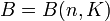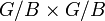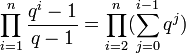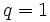# Difference between revisions of "Bruhat decomposition theorem"

## Statement

The Bruhat decomposition is a decomposition of a connected reductive linear algebraic group$G$ over a field$K$. We denote by$B$ a Borel subgroup of$G$ and by$W$ the Weyl group corresponding to a maximal torus contained in that Borel subgroup in$G$.

Note on algebraically closed: If$K$ is algebraically closed, then the Bruhat decomposition, up to isomorphism, depends only on the choice of$G$ and not on the specification of$B$ and$W$. This essentially follows from the Borel-Morozov theorem. If$K$ is not algebraically closed, we need to explicitly specify the$B$ and$W$ that are being used.

The standard case of interest is where$G = GL(n,K)$ denotes the general linear group: the group of invertible$n \times n$ matrices over$K$. Let$B = B(n,K)$ denote the Borel subgroup of$GL(n,K)$: the subgroup comprising the upper triangular invertible matrices. Let$W = S_n$ denote the symmetric group of degree$n$ viewed as a group of permutation matrices, viewed as matrices over$K$. Note that$S_n$ is a subgroup of$GL(n,K)$ when viewed this way.

### Double coset formulation

The elements of the Weyl group form representatives for the double coset space of$B$ in$G$.$G = \bigsqcup_{w \in W} BwB$.

### Partition of space of complete flags

The double cosets of$B$ in$G$ can be identified with the sets of left cosets that live in them. Converting the double coset formulation into this language, we have:$G/B = \bigsqcup_{w \in W} BwB/B$

where each$BwB/B$ can be thought of as the subset of the left coset space$G/B$.

In the case$G = GL(n,K)$ with the corresponding choices of$B$ and$W$, the set$G/B$ is identified with the set of complete flags of subspaces in$n$-dimensional space over$k$.

### Formulation in terms of actions

Consider the left coset space$G/B$.$G$ acts on this by left multiplication. Now, consider the induced diagonal action on$G/B \times G/B$. Further, since$B$ is a subgroup of$G$, we get a corresponding action of$B$ on$G/B \times G/B$ by left multiplication.

The claim is that the orbits under this action can be identified with the elements of$W$. More explicitly, each orbit has a unique representative of the form:$(1,w), w \in W$

Here, 1 stands for the identity element of$W$.

Further, every element of the above form occurs in exactly one orbit.

## Combinatorics

The combinatorial version of the Bruhat decomposition theorem considers:$G/B = \bigsqcup_{w \in W} BwB/B$

and identifies each of the pieces$BwB/B$ as a Schubert cell.

For the case that$K$ is a finite field of size$q$ and we are considering$G = GL(n,K)$, the size of each cell is a polynomial in$q$. The Weyl group$W$ is the symmetric group$S_n$. The polynomial itself depends only on the corresponding element of$S_n$ and not on$q$. In fact, it turns out that this polynomial is just$q^d$ where$d$ is the Bruhat word length. The sum of all these polynomials is the polynomial giving the size of$G/B$, which is:$\prod_{i=1}^n \frac{q^i - 1}{q - 1} = \prod_{i=2}^n (\sum_{j=0}^{i-1} q^j)$

Plugging in$q = 1$ in this expression gives$n!$, the order of the symmetric group.

## Particular cases

### General linear group

Value of$n$ Corresponding symmetric group$S_n$ Corresponding general linear group$GL(n,K)$ Discussion of Bruhat decomposition
1 trivial group multiplicative group of$K$ only one piece, not interesting
2 cyclic group:Z2 general linear group of degree two Bruhat decomposition for general linear group of degree two over a field
3 symmetric group:S3 general linear group of degree three Bruhat decomposition for general linear group of degree three over a field
4 symmetric group:S4 general linear group of degree four Bruhat decomposition for general linear group of degree four over a field